# Stirling interpolation formula

(diff) ← Older revision | Latest revision (diff) | Newer revision → (diff)

The half-sum of the Gauss interpolation formula for forward interpolation with respect to the nodesat the point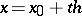: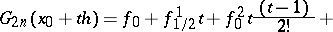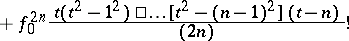and Gauss' formula of the same order for backward interpolation with respect to the nodes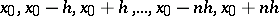: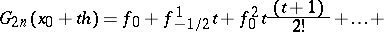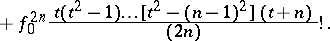Using the notation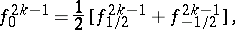Stirling's interpolation formula takes the form: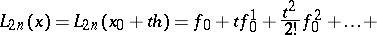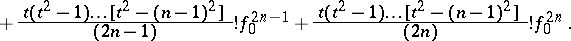For small, Stirling's interpolation formula is more exact than other interpolation formulas.

How to Cite This Entry:
Stirling interpolation formula. Encyclopedia of Mathematics. URL: http://encyclopediaofmath.org/index.php?title=Stirling_interpolation_formula&oldid=12181
This article was adapted from an original article by M.K. Samarin (originator), which appeared in Encyclopedia of Mathematics - ISBN 1402006098. See original article